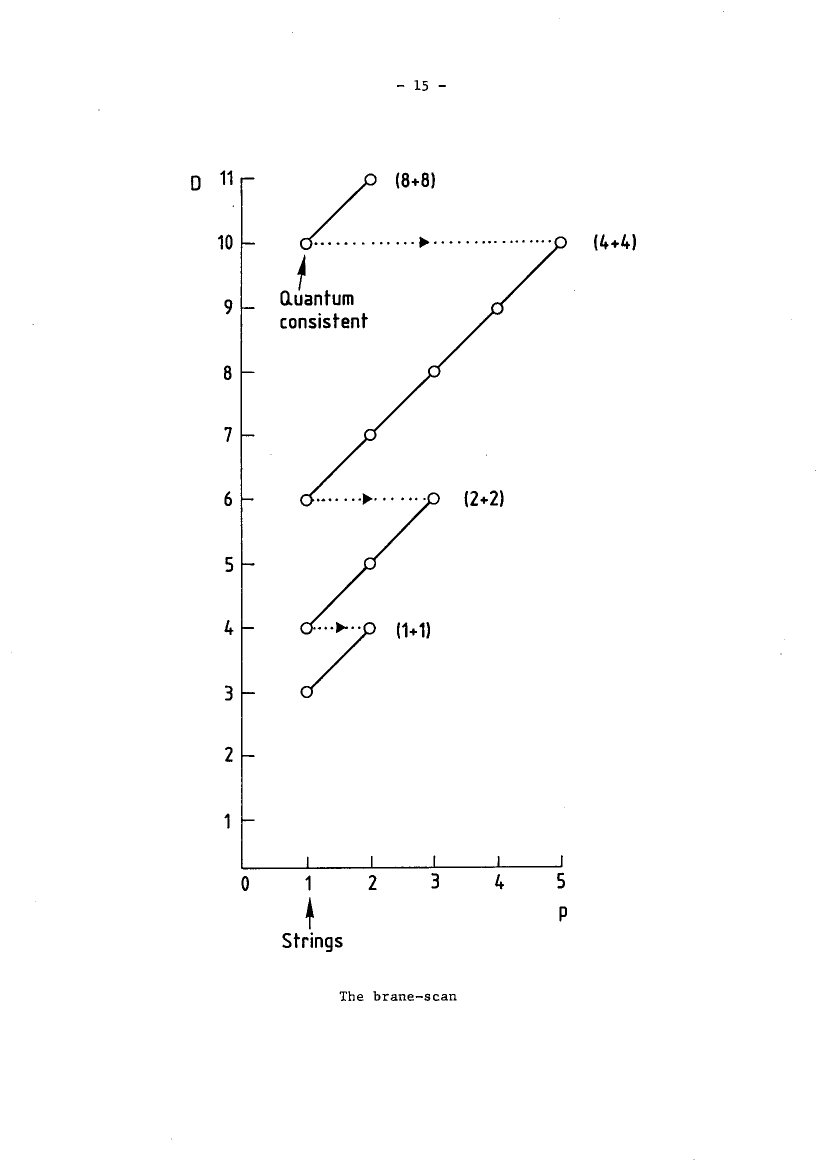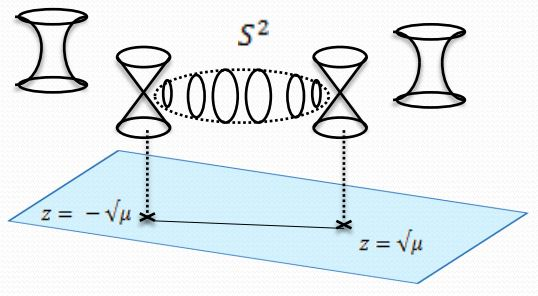Contents

# Contents

## Idea

What is called double dimensional reduction is a variant of Kaluza-Klein mechanism combined with fiber integration in the presence of branes: given a spacetime of dimension $d+1$ in which a $p+1$-brane propagates, its KK-reduction results in a $d$-dimensional effective spacetime containing a $p+1$-brane together with a “doubly reduced” $p$-brane, which is the reduction of those original $(p+1)$-brane configurations that wrapped the cycle along which the KK-reduction takes place.

## Definition

### Via fiber integration in ordinary differential cohomology

Let $\mathbf{H}$ be the smooth topos. For $p+1 \in \mathbb{N}$ write $\mathbf{B}^{p+1}U(1)_{conn} \in \mathbf{H}$ for the universal moduli stack of circle n-bundles with connection (given by the Deligne complex).

Notice that fiber integration in ordinary differential cohomology has the following stacky incarnation (see here):

###### Proposition

For $\Sigma$ an oriented closed manifold of dimension $k \leq p+1$, then fiber integration in ordinary differential cohomology is reflected by a morphism of the form

$\array{ [\Sigma, \mathbf{B}^{p+1}U(1)_{conn}] &\stackrel{\int_\Sigma}{\longrightarrow}& \mathbf{B}^{p+1-k}U(1)_{conn} \\ \downarrow^{[\Sigma,curv]} && \downarrow^{curv} \\ [\Sigma,\mathbf{\Omega}^{p+2}] &\stackrel{\int_\Sigma}{\longrightarrow}& \mathbf{\Omega}^{p+2-k} } \,,$

where the vertical morphisms are the curvature maps and the bottom morphisms reflects ordinary fiber integration of differential forms.

###### Definition

Given a cocycle

$\nabla \;\colon\; X \times \Sigma \longrightarrow \mathbf{B}^{p+1}U(1)_{conn} \,,$

on the Cartesian product of some smooth space $X$ with $\Sigma$, then its double dimensional reduction is the cocycle on $X$ which is given by the composite

$X \longrightarrow [\Sigma, X \times \Sigma] \stackrel{[\Sigma,\nabla]}{\longrightarrow} [\Sigma,\mathbf{B}^{p+1}U(1)_{conn}] \stackrel{\int_\Sigma}{\longrightarrow} \mathbf{B}^{p+1-k}U(1)_{conn} \,,$

where the first morphism is the unit of the (Cartesian product $\dashv$ internal hom)-adjunction.

### Via cyclic loop spaces

We discuss here a formalization of double dimensional reduction via cyclification adjunction (FSS 16, section 3, BMSS 18, section 2.2). For more see at geometry of physics – fundamental super p-branes the section on double dimensional reduction.

###### Proposition

Let $\mathbf{H}$ be any (∞,1)-topos and let $G$ be an ∞-group in $\mathbf{H}$. Then the right base change/dependent product along the canonical point inclusion $\ast \to \mathbf{B}G$ into the delooping of $G$ takes the following form: There is a pair of adjoint ∞-functors of the form

$\mathbf{H} \underoverset {\underset{[G,-]/G}{\longrightarrow}} {\overset{hofib}{\longleftarrow}} {\bot} \mathbf{H}_{/\mathbf{B}G} \,,$

where

• $[G,-]$ denotes the internal hom in $\mathbf{H}$,

• $[G,-]/G$ denotes the homotopy quotient by the conjugation ∞-action for $G$ equipped with its canonical ∞-action by left multiplication and the argument regarded as equipped with its trivial $G$-$\infty$-action (for $G = S^1$ the circle group this is the cyclic loop space construction).

Hence for

then there is a natural equivalence

$\underset{ \text{original} \atop \text{fluxes} }{ \underbrace{ \mathbf{H}(\hat X\;,\; A) } } \;\; \underoverset {\underset{oxidation}{\longleftarrow}} {\overset{reduction}{\longrightarrow}} {\simeq} \;\; \underset{ \text{doubly} \atop { \text{dimensionally reduced} \atop \text{fluxes} } }{ \underbrace{ \mathbf{H}_{/\mathbf{B}G}(X \;,\; [G,A]/G) } }$

given by

$\left( \hat X \longrightarrow A \right) \;\;\; \leftrightarrow \;\;\; \left( \array{ X && \longrightarrow && [G,A]/G \\ & \searrow && \swarrow \\ && \mathbf{B}G } \right)$
###### Proof

First observe that the conjugation action on $[G,X]$ is the internal hom in the (∞,1)-category of $G$-∞-actions $Act_G(\mathbf{H})$. Under the equivalence of (∞,1)-categories

$Act_G(\mathbf{H}) \simeq \mathbf{H}_{/\mathbf{B}G}$

(from NSS 12) then $G$ with its canonical ∞-action is $(\ast \to \mathbf{B}G)$ and $X$ with the trivial action is $(X \times \mathbf{B}G \to \mathbf{B}G)$.

Hence

$[G,X]/G \simeq [\ast, X \times \mathbf{B}G]_{\mathbf{B}G} \;\;\;\;\; \in \mathbf{H}_{/\mathbf{B}G} \,.$

Actually, this is the very definition of what $[G,X]/G \in \mathbf{H}_{/\mathbf{B}G}$ is to mean in the first place, abstractly.

But now since the slice (∞,1)-topos $\mathbf{H}_{/\mathbf{B}G}$ is itself cartesian closed, via

$E \times_{\mathbf{B}G}(-) \;\;\; \dashv \;\;\; [E,-]_{\mathbf{B}G}$

it is immediate that there is the following sequence of natural equivalences

\begin{aligned} \mathbf{H}_{/\mathbf{B}G}(Y, [G,X]/G) & \simeq \mathbf{H}_{/\mathbf{B}G}(Y, [\ast, X \times \mathbf{B}G]_{\mathbf{B}G}) \\ & \simeq \mathbf{H}_{/\mathbf{B}G}( Y \times_{\mathbf{B}G} \ast, \underset{p^\ast X}{\underbrace{X \times \mathbf{B}G }} ) \\ & \simeq \mathbf{H}( \underset{hofib(Y)}{\underbrace{p_!(Y \times_{\mathbf{B}G} \ast)}}, X ) \\ & \simeq \mathbf{H}(hofib(Y),X) \end{aligned}

Here $p \colon \mathbf{B}G \to \ast$ denotes the terminal morphism and $p_! \dashv p^\ast$ denotes the base change along it.

## Examples

### Reduction on the circle

###### Example

When $\Sigma = S^1$ is the circle, and we think of $X \times S^1$ as a spacetime of 11-dimensional supergravity, then $\nabla \colon X \times S^1 \to \mathbf{B}^3 U(1)_{conn}$ may represent the supergravity C-field as a cocycle in ordinary differential cohomology. Then its double dimensional reduction in the sense of def. is the differential cocycle representing the B-field on $X$, in the sense of string theory.

###### Remark

For $\Sigma = S^1$ a circle as in example , then the morphism $X \longrightarrow [\Sigma, X \times \Sigma]$ in def. sends each point of $X$ to the loop in $X\times S^1$ that winds identically around the copy of $S^1$ at that point. Hence in this case it would make sense to consider, more generally, for each $p \in \mathbb{Z}$ the “order $p$” double dimensional reduction, given by the operation where one instead considers the map that lets the loop wind $p$ times around the $S^1$.

The resulting double dimensional reduction is just $p$-times the original one, so in a sense nothing much is changed, but maybe it is suggestive that now we are looking at the space of $C_p$-fixed points of the free loop space (for $C_p$ the cyclic group of order $p$). In E-infinity geometry this fixed-point structure on the free loop spaces makes the derived function algebras – the topological Hochschild homology of the original function algebras – be cyclotomic spectra.

### For general super $p$-branes

Double dimensional reduction for the super-$p$-branes in $D$ dimensions which are described by the Green-Schwarz action functional corresponds to moving down and left the diagonals in the brane scan table of consistent such branes:In particular

### From M-branes to F-branes

from M-branes to F-branes: superstrings, D-branes and NS5-branes

M-theory on $S^1_A \times S^1_B$-elliptic fibrationKK-compactification on $S^1_A$type IIA string theoryT-dual KK-compactification on $S^1_B$type IIB string theorygeometrize the axio-dilatonF-theory on elliptically fibered-K3 fibrationduality between F-theory and heterotic string theoryheterotic string theory on elliptic fibration
M2-brane wrapping $S_A^1$double dimensional reduction $\mapsto$type IIA superstring$\mapsto$type IIB superstring$\mapsto$$\mapsto$heterotic superstring
M2-brane wrapping $S_B^1$$\mapsto$D2-brane$\mapsto$D1-brane$\mapsto$
M2-brane wrapping $p$ times around $S_A^1$ and $q$ times around $S_B^1$$\mapsto$$p$ strings and $q$ D2-branes$\mapsto$(p,q)-string$\mapsto$
M5-brane wrapping $S_A^1$double dimensional reduction $\mapsto$D4-brane$\mapsto$D5-brane$\mapsto$
M5-brane wrapping $S_B^1$$\mapsto$NS5-brane$\mapsto$NS5-brane$\mapsto$$\mapsto$NS5-brane
M5-brane wrapping $p$ times around $S_A^1$ and $q$ times around $S_B^1$$\mapsto$$p$ D4-brane and $q$ NS5-branes$\mapsto$(p,q)5-brane$\mapsto$
M5-brane wrapping $S_A^1 \times S_B^1$$\mapsto$$\mapsto$D3-brane$\mapsto$
KK-monopole/A-type ADE singularity (degeneration locus of $S^1_A$-circle fibration, Sen limit of $S^1_A \times S^1_B$ elliptic fibration)$\mapsto$D6-brane$\mapsto$D7-branes$\mapsto$A-type nodal curve cycle degeneration locus of elliptic fibration(Sen 97, section 2)SU-gauge enhancement
KK-monopole orientifold/D-type ADE singularity$\mapsto$D6-brane with O6-planes$\mapsto$D7-branes with O7-planes$\mapsto$D-type nodal curve cycle degeneration locus of elliptic fibration(Sen 97, section 3)SO-gauge enhancement
exceptional ADE-singularity$\mapsto$$\mapsto$$\mapsto$exceptional ADE-singularity of elliptic fibration$\mapsto$E6-, E7-, E8-gauge enhancement

(e.g. Johnson 97, Blumenhagen 10)

Formalization of double dimensional reduction is discussed in rational homotopy theory in

and in full homotopy theory in

Exposition is in

and further discussion in

### Reduction of membrane to string

The concept of double dimensional reduction was introduced, for the case of the reduction of the supermembrane in 11d to the Green-Schwarz superstring in 10d, in

The above “brane scan” table showing the double dimensional reduciton pattern of the super-$p$-branes given by the Green-Schwarz action functional (see there for more references on this) is taken from

• Michael Duff, Supermembranes: the first fifteen weeks CERN-TH.4797/87 (1987) (scan)

### Reduction of M5-brane to D4-brane

The double dimensional reduction of the M5-brane to the D4-brane: